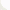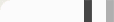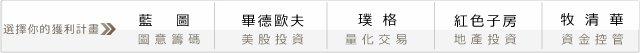## 程式獵人武器大對決－機關槍V.S手槍，談交易次數分析想必大家對城市獵人這部漫畫應該不陌生吧，

#### 回測時間，SHOW TIME!

(1)當短期（6根K棒）RSI線由下往上突破長期（12根K棒）RSI線時，

(2)到期當天出場，等到下次訊號出現時在進場，此方式可以避免到期換月問題。

1. 成本1000元，不加濾網（交易次數多）。
2. 成本2000元，不加濾網（交易次數多）。
3. 成本1000元，加濾網（交易次數少）。
4. 成本2000元，加濾網（交易次數少）。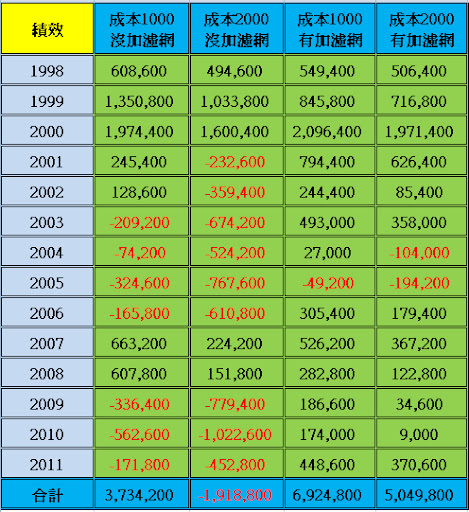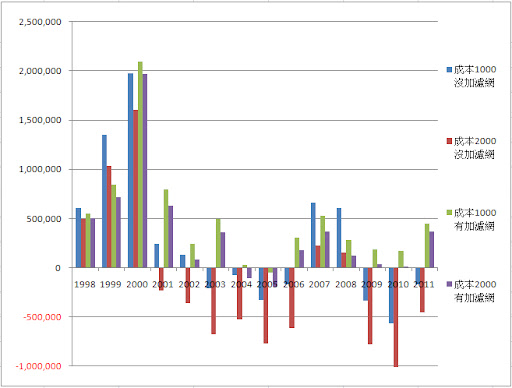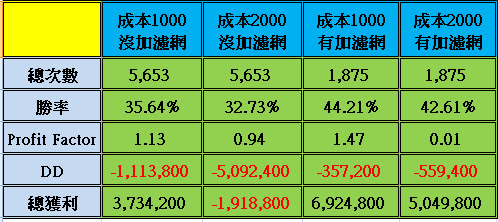if time=0915 then begin
dh=highd(0);
dl=lowd(0);
end;

if RSI(close,6) crosses above RSI (close,12) then begin
sellflag=false;
end;

if RSI(close,6) crosses below RSI (close,12) then begin
sellflag=true;
end;

if buyflag=true and high > dh and high > highest(h,3) then begin
buy next bar at highest(high,3)+1 stop;
end;

if sellflag=true and low< dl and low < lowest(l,3) then begin
sell next bar at lowest(low,3)-1 stop;
end;

if marketposition > 0 then exitlong at entryprice-150 stop ;
if marketposition < 0 then exitshort at entryprice+150 stop ;

Condition0=(dayofweek(date)=3 and dayofmonth(date)>14 and 22>dayofmonth(date)) or Date=1040127
or Date=1070226
or Date=1100617
or Date=1100222;
if condition0=true then begin
if t>1314 then begin
exitlong("EXPL") on close;
exitshort("EXPS") on close;
end;
end;

#### 0 意見: